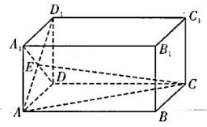$\text{A.}$ $12 \pi$ $\text{B.}$ $16 \pi$ $\text{C.}$ $20 \pi$ $\text{D.}$ $36 \pi$#### 解析：

①点击 首页查看更多试卷和试题 , 点击查看 本题所在试卷